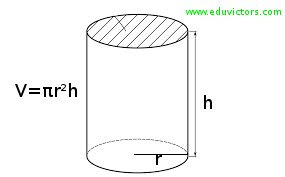Surface Areas and Volumes (Cylinder)

EXERCISE 13.6

Q1: The circumference of the base of a cylindrical vessel is 132 cm and its height is 25 cm. How many litres of water can it hold? (1000 cm³ = 1l)

Answer: Given, h = 25 cm, 2πr = 132 cm.
2πr = 132
2 × (22/7) × r = 132
r = (132 × 7)/ (2 × 22) = 21cm

Volume = πr²h = (22/7) × 21 × 21 × 25
= 34650 cm³
= 34650/1000

Q2: The inner diameter of a cylindrical wooden pipe is 24 cm and its outer diameter is 28 cm. The length of the pipe is 35 cm. Find the mass of the pipe, if 1 cm³ of wood has a mass of 0.6 g.

Outer radius (R) = 28/2 = 14cm
h = 35 cm
Volume of the wood used in the pipe = π(R² – r²) h
= (22/7) × (14² – 12²) × 35
= (22/7) × 26 × 2 × 35
= 5720 cm³

Mass of 1 cm³ of wood = 0.6 g
∴ Mass of 5720 cm³ of wood = 0.6 × 5720 g = 3432 g = 3.432 kg (Answer)

Q3: A soft drink is available in two packs —
(i) a tin can with a rectangular base of length 5 cm and width 4 cm, having a height of 15 cm and
(ii) a plastic cylinder with circular base of diameter 7 cm and height 10 cm.

Which container has greater capacity and by how much?

For tin can with rectangular base (cuboid).
Given,
l = 5 cm, b = 4 cm, h = 15 cm
Volume of the tin can = lbh = 5 × 4 × 15 = 300 cm³

For plastic cylinder with circular base.
Given,
r = 7/2= 3.5cm,
h = 10 cm
Volume of the plastic cylinder = πr²h
= (22/7) × 3.5 × 3.5 × 10  = 385 cm³

Difference in the capacities of the two containers = (385 – 300) cm3 = 85 cm³
Thus, the plastic cylinder with circular base has greater capacity by 85 cm³ (Answer)

Q4: If the lateral surface of a cylinder is 94.2 cm² and its height is 5 cm, then find
(ii) its volume (Use π = 3.14)

Answer: Given LSA = 2πrh = 94.2 cm²
h = 5 cm,

(i) 2πrh = 94.2 cm²
2 × 3.14 × r × 5 = 94.2
r = 94.2 / (2 × 3.14 × 5) = 3cm (Answer)

(ii) Volume of the cylinder = πr²h
= 3.14 × 3 × 3 × 5  = 141.3 cm³ (Answer)

Q5: It costs Rs 2200 to paint the inner curved surface of a cylindrical vessel 10m deep. If the cost of painting is at the rate of Rs 20 per m², find

(i) Inner curved surface area of the vessel,
(iii) capacity of the vessel.

Answer: Given, h = 10 m
(i) Inner curved surface area =

 CSA = Total cost Cost of painting per m²

(ii) Since, 2πrh = 110
⇒ 2 × (22/7) × r × 10 = 110
⇒ r = (110 × 7) / (2 × 22 × 10)

(iii) Capacity of the vessel = πr²h
= (22/7) × 1.75 × 1.75 × 10  = 96.25 m³
= 96.25 kl (Answer) [1 m³ = 1 kl]

Q6: The capacity of a closed cylindrical vessel of height 1 m is 15.4 litres. How many square metres of metal sheet would be needed to make it?

Answer: Given, h = 1 m, volume = 15.4 litres
= 15.4 / 1000 = 0.0154 m³
Vol. of Cylinder =  πr²h = 0.0154 m³
⇒ r² = (0.0154 × 7) / 22 = 0.0049
⇒ r = 0.07m

∴ Total surface area of the cylinder = 2πr (h + r)
= 2 × (22/7) × 0.07 (1 + 0.07) m²
= 44 × 0.01 × 1.07 m2 = 0.4708 m² (Answer)

Q7: A lead pencil consists of a cylinder of wood with a solid cylinder of graphite filled in the interior. The diameter of the pencil is 7 mm and the diameter of the graphite is 1 mm. If the length of the pencil is 14 cm, find the volume of the wood and that of the graphite.

Answer: Given, h = 14 cm.
Radius of the pencil (R) = 7/2 = 3.5mm = 0.35 cm.
Radius of the graphite (r) = ½ mm = 0.05 cm.
Volume of the the graphite = πr²h
= (22/7) × 0.05 × 0.05 × 14 = 0.11 cm³

Volume of the the wood = π (R² – r²)h
= (22/7) × [(0.35)² – (0.05)²] × 14 cm³
= (22/7) × 0.4 × 0.3 × 14 cm³ = 5.28 cm³

Thus, volume of the wood = 5.28 cm³ and volume of the graphite = 0.11 cm³ (Answer).

Q8: A patient in a hospital is given soup daily in a cylindrical bowl of diameter 7 cm. If the bowl is filled with soup to a height of 4 cm, how much soup the hospital has to prepare daily to serve 250 patients?

Given, r = 7/2 = 3.5 cm, h = 4 cm
Capacity of 1 cylindrical bowl =  πr²h
= (22/7) × 3.5 × 3.5 × 4 = 154 cm³

Thus, soup consumed by 250 patients per day = 250 × 154 cm³ = 38500 cm³ (Answer)